Students can download Maths Chapter 4 Geometry Ex 4.7 Questions and Answers, Notes, Samacheer Kalvi 9th Maths Guide Pdf helps you to revise the complete Tamilnadu State Board New Syllabus, helps students complete homework assignments and to score high marks in board exams.

## Tamilnadu Samacheer Kalvi 9th Maths Solutions Chapter 4 Geometry Ex 4.7

Multiple Choice Questions

Question 1.
The exterior angle of a triangle is equal to the sum of two ……….
(a) Exterior angles
(b) Interior opposite angles
(c) Alternate angles
(d) Interior angles
Solution:
(b) Interior opposite anglesQuestion 2.
In the quadrilateral ABCD, AB = BC and AD = DC Measure of ∠BCD is …………..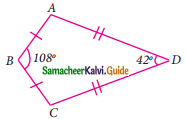(a) 150°
(b) 30°
(c) 105°
(d) 72°
Solution:
(c) 105°
Hint:
Join BD
∠DBC = 54°; ∠BDC = 21°
∴ ∠BCD = 180° – (54° + 21°)
= 180° – 75°
= 105°Question 3.
ABCD is a square, diagonals AC and BD meet at O. The number of pairs of congruent triangles with vertex O are ……….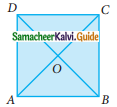(a) 6
(b) 8
(c) 4
(d) 12
Solution:
(a) 6

Question 4.
In the given figure CE || DB then the value of x° is ………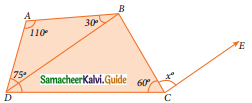(a) 45°
(b) 30°
(c) 75°
(d) 85°
∠B = 360° – (∠A + ∠D + ∠BCD)
= 360° – (110° + 75° + 60°)
= 360° – 245°
= 115°
∠DBC = 115° – 30°
= 85°
∴ ∠x = 85° (BD || CE) Alternate angles are equalQuestion 5.
The correct statement out of the following is ……..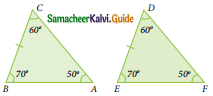(a) ΔABC ≅ ΔDEF
(b) ΔABC ≅ ΔEDF
(c) ΔABC ≅ ΔFDE
(d) ΔABC ≅ ΔFED
Solution:
(d) ΔABC ≅ ΔFED

Question 6.
If the diagonal of a rhombus are equal, then the rhombus is a ……..
(a) Parallelogram but not a rectangle
(b) Rectangle but not a square
(c) Square
(d) Parallelogram but not a square
Solution:
(c) SquareQuestion 7.
If bisectors of ∠A and ∠B of a quadrilateral ABCD meet at O, then ∠AOB is ……..
(a) ∠C + ∠D
(b) $$\frac{1}{2}$$ (∠C + ∠D)
(c) $$\frac{1}{2}$$ ∠C + $$\frac{1}{3}$$ ∠D
(d) $$\frac{1}{3}$$ ∠C + $$\frac{1}{2}$$ ∠D
Solution:
(b) $$\frac{1}{2}$$ (∠C + ∠D)

Question 8.
The interior angle made by the side in a parallelogram is 90° then the parallelogram is a …….
(a) rhombus
(b) rectangle
(c) trapezium
(d) kite
Solution:
(b) rectangle
Hint:
Opposite sides are equal and each angle is 90° then it is a rectangle.Question 9.
Which of the following statement is correct?
(a) Opposite angles of a parallelogram are not equal
(b) Adjacent angles of a parallelogram are complementary.
(c) Diagonals of a parallelogram are always equal.
(d) Both pairs of opposite sides of a parallelogram are always equal.
Solution:
(d) Both pairs of opposite sides of a parallelogram are always equal.

Question 10.
The angles of the triangle are 3x – 40, x + 20 and 2x – 10 then the value of x is ………
(a) 40°
(b) 35°
(c) 50°
(d) 45°
Solution:
(b) 35°
Hint:
3x – 40 + x + 20 + 2x – 10 = 180° (Sum of the angles of a triangle is 180°)
6x – 30 = 180°
6x = 180°+ 30°
x = $$\frac{210°}{6°}$$
= 35°Question 11.
PQ and RS are two equal chords of a circle with centre O such that ∠POQ = 70°, then ∠ORS = ……….
(a) 60°
(b) 70°
(c) 55°
(d) 80°
Solution:
(c) 55°
Hint: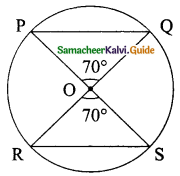∠POQ = 70° (Vertically opposite Angle)
∠ORS and ∠OSR are equal. (OR = OS radius of the circle)
∠ORS + ∠OSR + ∠ROS = 180°
x° + x° + 70° = 180°
2x + 70° = 180°
2x = 180° – 70° = 110°
x = $$\frac{110°}{2}$$ = 55°
∴ ∠ORS = 55°Question 12.
A chord is at a distance of 15cm from the centre of the circle of radius 25cm. The length of the chord is ……….
(a) 25cm
(b) 20cm
(c) 40cm
(d) 18cm
Solution:
(c) 40cm
Hint: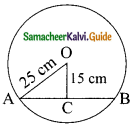In the right triangle OAC,
AC² = OA² – OC²
= 25² – 15²
= (25 + 15) (25 – 15)
= 40 × 10
AC² = 400
AC = $$\sqrt{400}$$
= 20
Length of the chord AB = 20 + 20 = 40 cm.Question 13.
In the figure, O is the centre of the circle and ∠ACB = 40° then ∠AOB = ………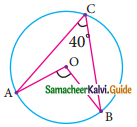(a) 80°
(b) 85°
(c) 70°
(d) 65°
Solution:
(a) 80°
Hint:
∠AOB = 2∠ACB (The angle subtended by an arc of the circle at the centre is double the angle subtended at the remaining part of the circle.)
= 2 × 40° = 80°

Question 14.
In a cyclic quadrilaterals ABCD, ∠A = 4x, ∠C = 2x the value of x is ……..
(a) 30°
(b) 20°
(c) 15°
(d) 25
Solution:
(a) 30°
Hint: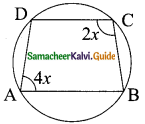∠A + ∠C = 180° (Sum of the opposite angle of cyclic quadrilateral is 180°)
4x + 2x = 180°
x = $$\frac{180°}{6}$$
= 30°Question 15.
In the figure, O is the centre of a circle and diameter AB bisects the chord CD at a point E such that CE = ED = 8 cm and EB = 4cm. The radius of the circle is ………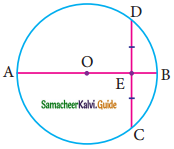(a) 8cm
(b) 4cm
(c) 6cm
(d) 10cm
Solution:
(i) 10cm
Hint: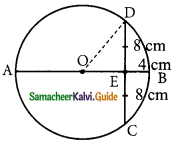Let the radius OD be x.
OE = OB – BE
= x – 4 (OB radius of the circle)
In the ΔOED,
OD² = OE² + ED²
x² = (x – 4)² + 8²
x² = x² + 16 – 8x + 64
8x = 80
x = $$\frac{80}{8}$$
= 10 cm
Radius of the circle = 10 cm.Question 16.
In the figure, PQRS and PTVS are two cyclic quadrilaterals, If ∠QRS = 80°, then ∠TVS = ………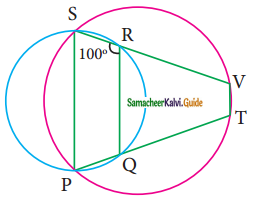(a) 80°
(b) 100°
(c) 70°
(d) 90°
Solution:
(a) 80°
Hint:
∠SPQ = 180° – 80° (Opposite angles of a cyclic quadrilateral PQRS)
= 100°
∠V = 180° – 100° = 80° (Opposite angles of a cyclic quadrilateral)

Question 17.
If one angle of a cyclic quadrilateral is 75°, then the opposite angle is ………
(a) 100°
(b) 105°
(c) 85°
(d) 90°
Solution:
(b) 105°
Hint:
Opposite angles = 180° – 75°
= 105° (Sum of the opposite angle is 180°)Question 18.
In the figure, ABCD is a cyclic quadrilateral in which DC produced to E and CF is drawn parallel to AB such that ∠ADC = 80° and ∠ECF = 20°, then BAD = ?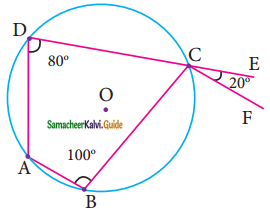(a) 100°
(b) 20°
(c) 120°
(d) 110°
Solution:
(c) 120°
Hint:
∠BAD = ∠ABC + ∠ECF (By exterior angle property)
= 100° + 20°
= 120°Question 19.
AD is a diameter of a circle and AB is a chord If AD = 30cm and AB = 24cm then the distance of AB from the centre of the circle is ………
(a) 10cm
(b) 9cm
(c) 8cm
(d) 6cm
Solution:
(b) 9cm
Hint: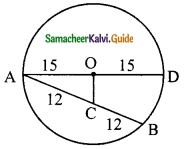In ΔAOC,
AO = 15 cm
AC = $$\frac{1}{2}$$ AB
= $$\frac{1}{2}$$ × 24
= 12 cm
In ΔAOC,
OC² = AO² – AC²
= 15² – 12²
= 225 – 144
= 81
OC = $$\sqrt{81}$$
= 9 cmQuestion 20.
In the given figure, If OP = 17cm, PQ = 30cm and OS is perpendicular to PQ, then RS is ……….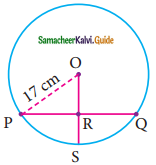(a) 10cm
(b) 6cm
(c) 7cm
(d) 9cm
Solution:
(d) 9cm
Hint:
In ΔOPR, OP = 17cm, PR = 30/2 = 15 cm.
OR² = OP² – PR²
= 17² – 15²
= (17 + 15) (17 – 15)
= 32 × 2
OR² = $$\sqrt{64}$$
= 8 cm
RS = OS – OR
= 17 – 8
= 9 cm